# NCERT Solutions for Class 8 Maths Chapter 3 Understanding Quadrilaterals Ex 3.3

These NCERT Solutions for Class 8 Maths Chapter 3 Understanding Quadrilaterals Ex 3.3 Questions and Answers are prepared by our highly skilled subject experts.

## NCERT Solutions for Class 8 Maths Chapter 3 Understanding Quadrilaterals Exercise 3.3

Question 1.
Given a parallelogram ABCD. Complete each statement along with the definition or property used.(ii) ∠DCB = __________
(iii) OC = __________
(iv) m∠DAB + m∠CDA = __________
Solution:
(i) AD = BC (opposite sides are equal)
(ii) ∠DCB = ∠DAB (opposite angles are equal)
(iii) OC = OA (Diagonals bisect each other)
(iv) m∠DAB + m∠CDA = 180° (Adjacent angles are supplementary)Question 2.
Consider the following parallelograms. Find the values of the unknown x, y, z.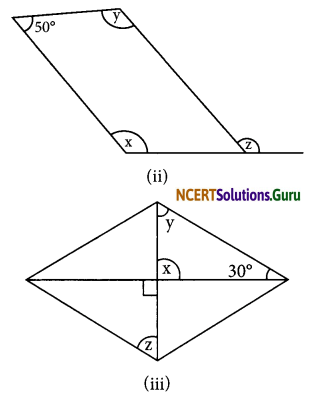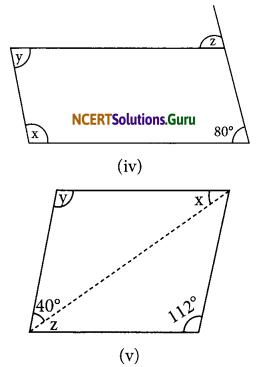Solution:
(i) ∠y = 100° (opposite angles of a parallelogram are equal)∠x + 100° = 180° (adjacent angles in a parallelogram)
∠x = 180° – 100° = 80°
∠z = ∠x = 80° (opposite angles of parallelogram are equal)
∴ ∠x = 80°, ∠y = 100°, ∠z = 80°

(ii) Opposite angles are equal.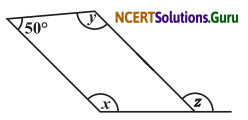∠1 = 50° (opposite angles are equal)
∠1 + ∠z = 180° (Linear pair)
50 + ∠z = 180
∠z = 180° – 50°= 130°
x + ∠1 + y + 50° = 360°
⇒ x + 50° + y + 50° = 360°
⇒ x + y + 100° = 360°
⇒ x + y = 360° – 100°
⇒ x + y = 260°
∴ x = y (opposite angles of a parallelogram)
x = $$\frac{260^{\circ}}{2}$$ = 130°
Thus x = 130°, y = 130° and z = 130°.(iii) x = 90° (vertically opposite angles are equal)x + y + 30° = 180°
(sum of the angles of a triangle = 180°)
90° + y + 30° = 180°
y + 120° = 180°
∠y = 180° – 120° = 60°
In the parallelogram ABCD
AD || BC and BD is a transversal.
∴ y = z (alternate angles are equal)
z = y = 60°
Thus x = 90°, y = 60° and z = 60°

(iv) In the parallelogram ABCD
y = 80° (opposite angles are equal)
AD || BC and CD is a transversal equal)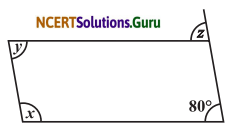∴ z = 80° (corresponding angles are equal)
x + 80° = 180° (sum of the adjacent angle is 180°)
x = 180° – 80° = 100°
Thus, x = 100°, y = 80° and z = 80°

(v) y = 112° (in a parallelogram, opposite angles are equal)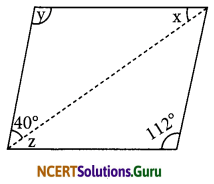In ΔACD,
x + y + 40° = 180° (sum of the angles of a triangle is 180°)
⇒ x + 112° + 40° = 180°
⇒ x + 152°= 180°
⇒ x = 180° – 152° = 28°
∠C + ∠B = 180° (sum of the adjacent angles of a parallelogram is 180°)
⇒ 40° + z + 112° = 180°
⇒ z + 152° = 180°
⇒ z = 180° – 152° = 28°
Thus, x = 28°, y = 112° and z = 28°.Question 3.
Can a quadrilateral ABCD be a parallelogram if
(i) ∠D + ∠B = 180°?
(ii) AB = DC = 8 cm, AD = 4 cm and BC = 4.4 cm?
(iii) ∠A = 70° and ∠C = 65°?
Solution:
∠D + ∠B = 180° can be, but need not be
∴ The quadrilateral may be a parallelogram but not always

AB = DC = 8 cm
BC = 4.4 cm
∴ Opposite sides AD and BC are not equal.
∴ It cannot be a parallelogram.

∠A = 70° and ∠C = 65°
∵ Opposite angles ∠A ≠ ∠C
∴ It cannot be a parallelogram.

Question 4.
Draw a rough figure of a quadrilateral that is not a parallelogram but has exactly two opposite angles of equal measure.
Solution:
In the adjoining figure, ABCD is not a parallelogram such that opposite angles ∠B and ∠D are equal. It is a kite.Question 5.
The measures of two adjacent angles of a parallelogram are in the ratio 3 : 2. Find the measure of each of the angles of the parallelogram.
Solution:
Let ABCD be a parallelogram in which adjacent angles ∠A and ∠B are 3x and 2x respectively.
∴ ∠A + ∠B = 180°
⇒ 3x + 2x = 180°
⇒ 5x = 180°
⇒ x = $$\frac{180^{\circ}}{5}$$ = 36°
∠A = 3 × 36° = 108°
and ∠B = 2 × 36° = 72°
Since opposite angles are equal.
∴ ∠D = ∠B = 72° and ∠C = ∠A = 108°
∴ ∠A = 108°, ∠B = 72°, ∠C = 108° and ∠D = 72°

Question 6.
Two adjacent angles of a parallelogram have equal measure. Find the measure of each of the angles of the parallelogram.
Solution:
Let ABCD be a parallelogram such that adjacent angles ∠A = ∠B
Since ∠A + ∠B = 180°
∠A = ∠B = $$\frac{180^{\circ}}{2}$$ = 90°
Since opposite angles of a parallelogram are equal
∴ ∠A = ∠C = 90° and ∠B = ∠D = 90°
Thus, ∠A = 90°, ∠B = 90°, ∠C = 90° and ∠D = 90°Question 7.
The adjacent figure HOPE is a parallelogram. Find the angle measures x, y and z. State the properties you use to find them.Solution:
y + z = 70°
(An exterior angles of a triangle is equal to the sum of the opposite interior angles)
∵ In ∆HOP
∠HOP = 180° – 70° = 110° (sum of the adjacent angle is 180°)
Now, x = ∠HOP = 110° (opposite angles of a parallelogram are equal)
EH || OP and PH is a transversal
∴ y = 40° (alternate angles are equal)
From (1),
y + z = 70°
⇒ 40° + z = 70°
⇒ z = 70° – 40° = 30°
Thus x = 110°, y = 40° and z = 30°

Question 8.
The following figures GUNS and RUNS are parallelograms. Find x and y. (Lengths are in cm)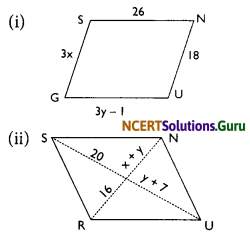Solution:
(i) GUNS is a parallelogram.
GS = NU (opposite sides are equal)
3x = 18
⇒ x = $$\frac{18}{3}$$ = 6
In the parallelogram GUNS
GU = SN (opposite sides are equal)
⇒ 3y – 1 = 26
⇒ 3y = 26 + 1
⇒ 3y = 27
⇒ y = $$\frac{27}{3}$$ = 9
Thus, x = 6 cm and y = 9 cm.(ii) RUNS is a parallelogram and the di-agonal RN and US bisect each other.
∴ y + 7 = 20
⇒ y = 20 – 7 = 13
x + y = 16
⇒ x + 13 = 16
⇒ x = 16 – 13 = 3
Thus, x = 3 cm and y = 13 cm

Question 9.
In the figure given below both RISK and CLUE are parallelograms. Find the value of x.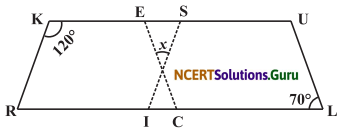Solution:
RISK is a parallelogram
∠R + ∠K = 180° (adjacent angles of a parallelogram are supplementary)
∴ ∠R + 120° = 180°
∠R = 180° – 120° = 60°
In the parallelogram RISK
∠R = ∠S (opposite angles are equal)
∠S = 60°
CLUE is also a parallelogram.
∠E = ∠L = 70° (opposite angle of a parallelogram)
∠E = 70°
Now, in triangle ESO
∠E + ∠S + x = 180°
⇒ 70° + 60° + x = 180°
⇒ 130° + x = 180°
⇒ x = 180° – 130°
⇒ x = 50°

Question 10.
Explain how this figure is a trapezium. Which of its two sides are parallel?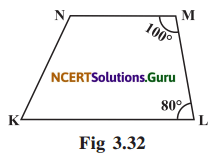Solution:
∠KLM + ∠NML = 80° + 100° = 180°
∴ KL || NM (The sum of consecutive interior angles is 180°)
∴ The given figure KLMN is a trapezium.Question 11.
Find m∠C in the figure given below if AB || DC.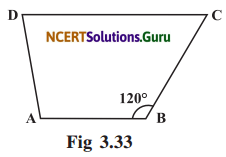Solution:
AB || DC and BC is a transversal,
∵ m∠B + m∠C = 180°
sum of interior angles is 180°
m∠C = 180° – m∠B
∴ m∠C = 180° – 120° = 60°

Question 12.
Find the measure of ∠P and ∠S if SP || QR in figure (if you find m∠R, is there more than one method to find m∠P)Solution:
PQRS is a trapezium such that SP || RQ and PQ is a transversal.
∴ m∠P + m∠Q = 180° (Interior angles are supplementary)
m∠P + 130° = 180°
m∠P = 180° – 130° = 50°
Also, m∠S + m∠R = 180°
⇒ m∠S + 90° = 180°
⇒ m∠S = 180° – 90°
⇒ m∠S = 90°
m∠P + m∠Q + m∠R + m∠S = 360° (sum of the angles of a quadrilateral is 360°)
⇒ m∠P + 130° + 90° + 90° = 360°
⇒ m∠P + 130° + 90° + 90° = 360°
⇒ m∠P + 130° + 90° + 90° = 360°
⇒ m∠P + 310° = 360°
⇒ m∠P = 360° – 310°
⇒ m∠P = 50°
Hence, m∠P = 50° and m∠S = 90°

error: Content is protected !!